Publicité

# PRESSURE Measurement

marcoReud
27 Oct 2022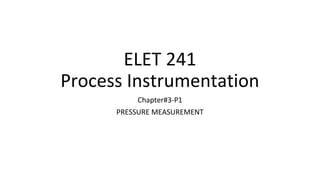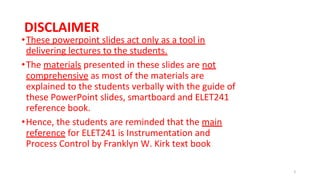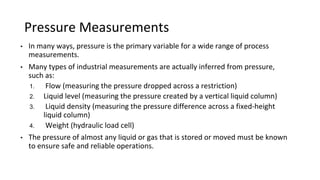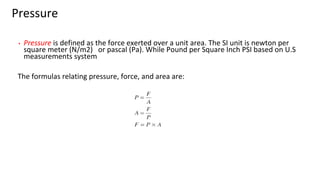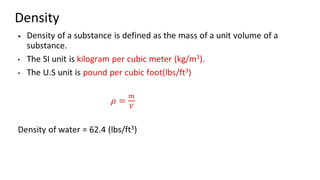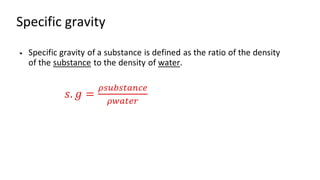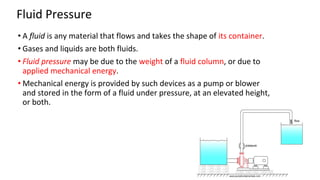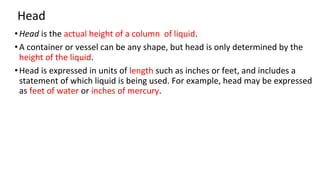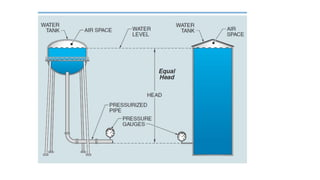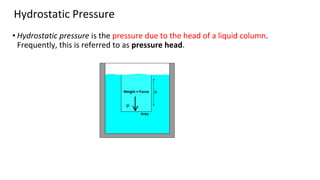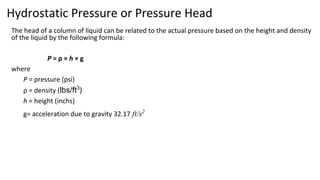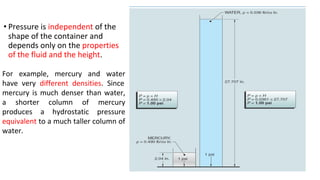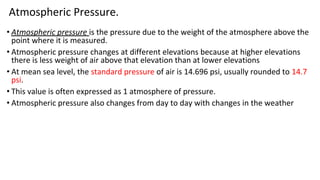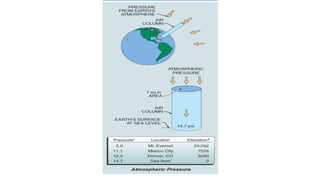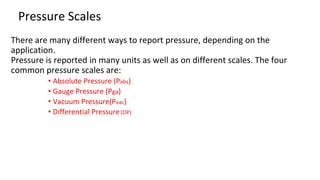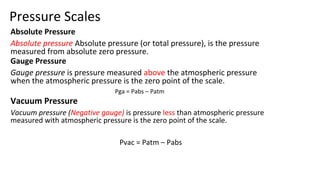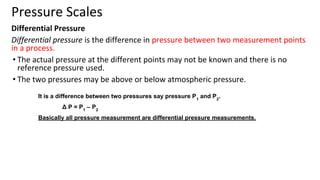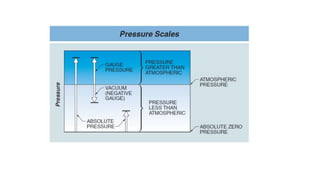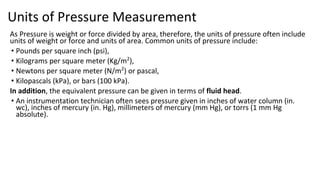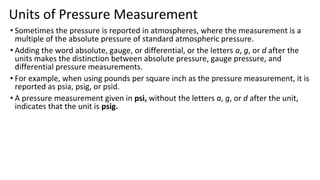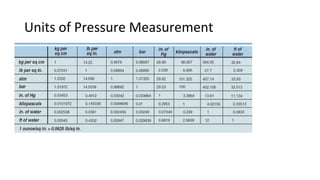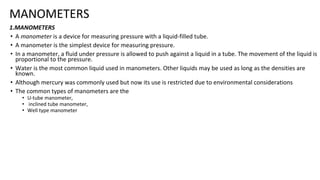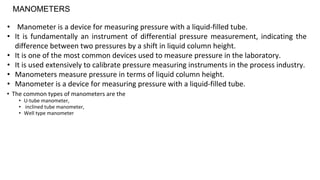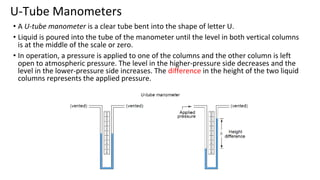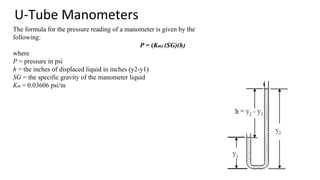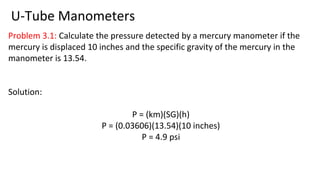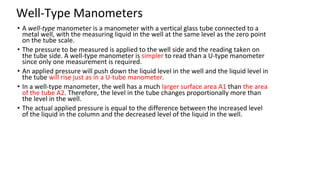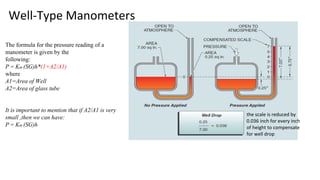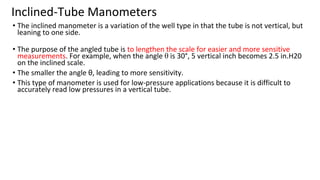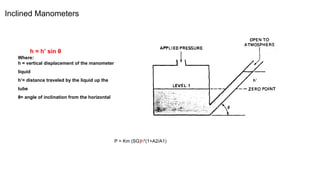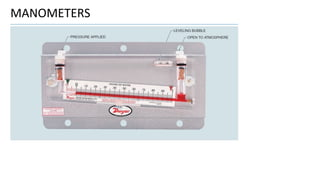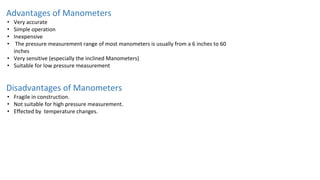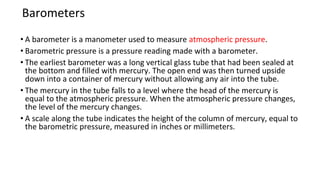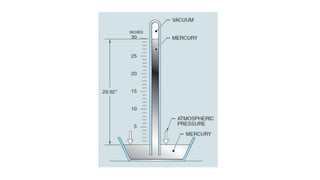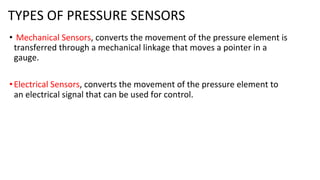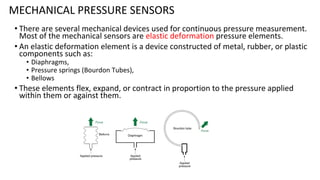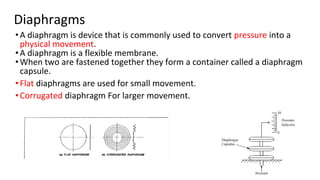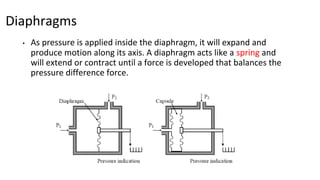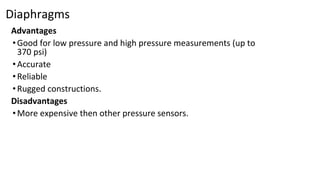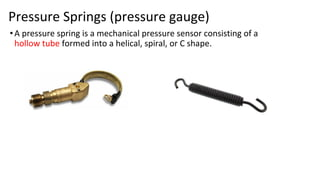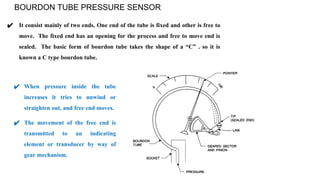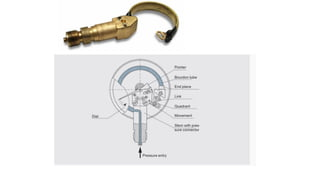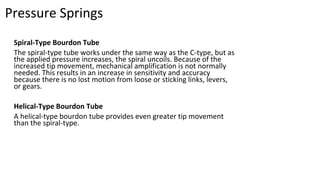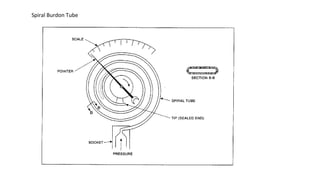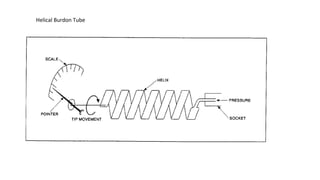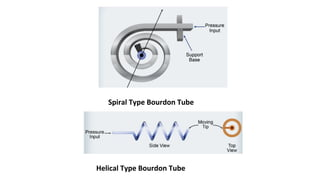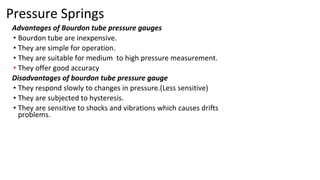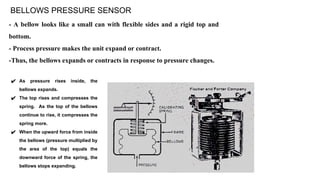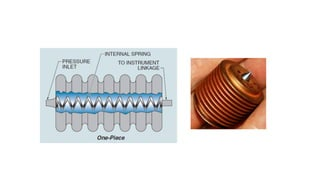1 sur 49
Publicité

### PRESSURE Measurement

1. ELET 241 Process Instrumentation Chapter#3-P1 PRESSURE MEASUREMENT
2. DISCLAIMER •These powerpoint slides act only as a tool in delivering lectures to the students. •The materials presented in these slides are not comprehensive as most of the materials are explained to the students verbally with the guide of these PowerPoint slides, smartboard and ELET241 reference book. •Hence, the students are reminded that the main reference for ELET241 is Instrumentation and Process Control by Franklyn W. Kirk text book 2
3. Pressure Measurements • In many ways, pressure is the primary variable for a wide range of process measurements. • Many types of industrial measurements are actually inferred from pressure, such as: 1. Flow (measuring the pressure dropped across a restriction) 2. Liquid level (measuring the pressure created by a vertical liquid column) 3. Liquid density (measuring the pressure difference across a fixed-height liquid column) 4. Weight (hydraulic load cell) • The pressure of almost any liquid or gas that is stored or moved must be known to ensure safe and reliable operations.
4. Pressure • Pressure is defined as the force exerted over a unit area. The SI unit is newton per square meter (N/m2) or pascal (Pa). While Pound per Square Inch PSI based on U.S measurements system The formulas relating pressure, force, and area are:
5. Density •
6. Specific gravity •
7. • A fluid is any material that flows and takes the shape of its container. • Gases and liquids are both fluids. • Fluid pressure may be due to the weight of a fluid column, or due to applied mechanical energy. • Mechanical energy is provided by such devices as a pump or blower and stored in the form of a fluid under pressure, at an elevated height, or both. Fluid Pressure
8. •Head is the actual height of a column of liquid. •A container or vessel can be any shape, but head is only determined by the height of the liquid. •Head is expressed in units of length such as inches or feet, and includes a statement of which liquid is being used. For example, head may be expressed as feet of water or inches of mercury. Head
9. Hydrostatic Pressure • Hydrostatic pressure is the pressure due to the head of a liquid column. Frequently, this is referred to as pressure head. h P
10. Hydrostatic Pressure or Pressure Head The head of a column of liquid can be related to the actual pressure based on the height and density of the liquid by the following formula: P = ρ × h × g where P = pressure (psi) ρ = density (lbs/ft3 ) h = height (inchs) g= acceleration due to gravity 32.17 ft/s2
11. • Pressure is independent of the shape of the container and depends only on the properties of the fluid and the height. For example, mercury and water have very different densities. Since mercury is much denser than water, a shorter column of mercury produces a hydrostatic pressure equivalent to a much taller column of water.
12. Atmospheric Pressure. • Atmospheric pressure is the pressure due to the weight of the atmosphere above the point where it is measured. • Atmospheric pressure changes at different elevations because at higher elevations there is less weight of air above that elevation than at lower elevations • At mean sea level, the standard pressure of air is 14.696 psi, usually rounded to 14.7 psi. • This value is often expressed as 1 atmosphere of pressure. • Atmospheric pressure also changes from day to day with changes in the weather
13. Pressure Scales There are many different ways to report pressure, depending on the application. Pressure is reported in many units as well as on different scales. The four common pressure scales are: • Absolute Pressure (Pabs) • Gauge Pressure (Pga) • Vacuum Pressure(Pvac) • Differential Pressure (DP)
14. Pressure Scales Absolute Pressure Absolute pressure Absolute pressure (or total pressure), is the pressure measured from absolute zero pressure. Gauge Pressure Gauge pressure is pressure measured above the atmospheric pressure when the atmospheric pressure is the zero point of the scale. Pga = Pabs – Patm Vacuum Pressure Vacuum pressure (Negative gauge) is pressure less than atmospheric pressure measured with atmospheric pressure is the zero point of the scale. Pvac = Patm – Pabs
15. Pressure Scales Differential Pressure Differential pressure is the difference in pressure between two measurement points in a process. • The actual pressure at the different points may not be known and there is no reference pressure used. • The two pressures may be above or below atmospheric pressure. It is a difference between two pressures say pressure P1 and P2 . Δ P = P1 – P2 Basically all pressure measurement are differential pressure measurements.
16. Units of Pressure Measurement As Pressure is weight or force divided by area, therefore, the units of pressure often include units of weight or force and units of area. Common units of pressure include: • Pounds per square inch (psi), • Kilograms per square meter (Kg/m2 ), • Newtons per square meter (N/m2 ) or pascal, • Kilopascals (kPa), or bars (100 kPa). In addition, the equivalent pressure can be given in terms of fluid head. • An instrumentation technician often sees pressure given in inches of water column (in. wc), inches of mercury (in. Hg), millimeters of mercury (mm Hg), or torrs (1 mm Hg absolute).
17. Units of Pressure Measurement • Sometimes the pressure is reported in atmospheres, where the measurement is a multiple of the absolute pressure of standard atmospheric pressure. • Adding the word absolute, gauge, or differential, or the letters a, g, or d after the units makes the distinction between absolute pressure, gauge pressure, and differential pressure measurements. • For example, when using pounds per square inch as the pressure measurement, it is reported as psia, psig, or psid. • A pressure measurement given in psi, without the letters a, g, or d after the unit, indicates that the unit is psig.
18. Units of Pressure Measurement
19. MANOMETERS 1.MANOMETERS • A manometer is a device for measuring pressure with a liquid-filled tube. • A manometer is the simplest device for measuring pressure. • In a manometer, a fluid under pressure is allowed to push against a liquid in a tube. The movement of the liquid is proportional to the pressure. • Water is the most common liquid used in manometers. Other liquids may be used as long as the densities are known. • Although mercury was commonly used but now its use is restricted due to environmental considerations • The common types of manometers are the • U-tube manometer, • inclined tube manometer, • Well type manometer
20. • Manometer is a device for measuring pressure with a liquid-filled tube. • It is fundamentally an instrument of differential pressure measurement, indicating the difference between two pressures by a shift in liquid column height. • It is one of the most common devices used to measure pressure in the laboratory. • It is used extensively to calibrate pressure measuring instruments in the process industry. • Manometers measure pressure in terms of liquid column height. • Manometer is a device for measuring pressure with a liquid-filled tube. • The common types of manometers are the • U-tube manometer, • inclined tube manometer, • Well type manometer MANOMETERS
21. U-Tube Manometers • A U-tube manometer is a clear tube bent into the shape of letter U. • Liquid is poured into the tube of the manometer until the level in both vertical columns is at the middle of the scale or zero. • In operation, a pressure is applied to one of the columns and the other column is left open to atmospheric pressure. The level in the higher-pressure side decreases and the level in the lower-pressure side increases. The difference in the height of the two liquid columns represents the applied pressure.
22. The formula for the pressure reading of a manometer is given by the following: P = (Km) (SG)(h) where P = pressure in psi h = the inches of displaced liquid in inches (y2-y1) SG = the specific gravity of the manometer liquid Km = 0.03606 psi/in U-Tube Manometers
23. Problem 3.1: Calculate the pressure detected by a mercury manometer if the mercury is displaced 10 inches and the specific gravity of the mercury in the manometer is 13.54. Solution: P = (km)(SG)(h) P = (0.03606)(13.54)(10 inches) P = 4.9 psi U-Tube Manometers
24. Well-Type Manometers • A well-type manometer is a manometer with a vertical glass tube connected to a metal well, with the measuring liquid in the well at the same level as the zero point on the tube scale. • The pressure to be measured is applied to the well side and the reading taken on the tube side. A well-type manometer is simpler to read than a U-type manometer since only one measurement is required. • An applied pressure will push down the liquid level in the well and the liquid level in the tube will rise just as in a U-tube manometer. • In a well-type manometer, the well has a much larger surface area A1 than the area of the tube A2. Therefore, the level in the tube changes proportionally more than the level in the well. • The actual applied pressure is equal to the difference between the increased level of the liquid in the column and the decreased level of the liquid in the well.
25. Well-Type Manometers the scale is reduced by 0.036 inch for every inch of height to compensate for well drop The formula for the pressure reading of a manometer is given by the following: P = Km (SG)h*(1+A2/A1) where A1=Area of Well A2=Area of glass tube It is important to mention that if A2/A1 is very small ,then we can have: P = Km (SG)h
26. Inclined-Tube Manometers • The inclined manometer is a variation of the well type in that the tube is not vertical, but leaning to one side. • The purpose of the angled tube is to lengthen the scale for easier and more sensitive measurements. For example, when the angle θ is 30°, 5 vertical inch becomes 2.5 in.H20 on the inclined scale. • The smaller the angle θ, leading to more sensitivity. • This type of manometer is used for low-pressure applications because it is difficult to accurately read low pressures in a vertical tube.
27. Inclined Manometers h = h’ sin θ Where: h = vertical displacement of the manometer liquid h’= distance traveled by the liquid up the tube θ= angle of inclination from the horizontal P = Km (SG)h*(1+A2/A1)
28. MANOMETERS
29. Advantages of Manometers • Very accurate • Simple operation • Inexpensive • The pressure measurement range of most manometers is usually from a 6 inches to 60 inches • Very sensitive (especially the inclined Manometers) • Suitable for low pressure measurement Disadvantages of Manometers • Fragile in construction. • Not suitable for high pressure measurement. • Effected by temperature changes.
30. Barometers • A barometer is a manometer used to measure atmospheric pressure. • Barometric pressure is a pressure reading made with a barometer. • The earliest barometer was a long vertical glass tube that had been sealed at the bottom and filled with mercury. The open end was then turned upside down into a container of mercury without allowing any air into the tube. • The mercury in the tube falls to a level where the head of the mercury is equal to the atmospheric pressure. When the atmospheric pressure changes, the level of the mercury changes. • A scale along the tube indicates the height of the column of mercury, equal to the barometric pressure, measured in inches or millimeters.
31. TYPES OF PRESSURE SENSORS • Mechanical Sensors, converts the movement of the pressure element is transferred through a mechanical linkage that moves a pointer in a gauge. •Electrical Sensors, converts the movement of the pressure element to an electrical signal that can be used for control.
32. MECHANICAL PRESSURE SENSORS • There are several mechanical devices used for continuous pressure measurement. Most of the mechanical sensors are elastic deformation pressure elements. • An elastic deformation element is a device constructed of metal, rubber, or plastic components such as: • Diaphragms, • Pressure springs (Bourdon Tubes), • Bellows • These elements flex, expand, or contract in proportion to the pressure applied within them or against them.
33. Diaphragms •A diaphragm is device that is commonly used to convert pressure into a physical movement. •A diaphragm is a flexible membrane. •When two are fastened together they form a container called a diaphragm capsule. •Flat diaphragms are used for small movement. •Corrugated diaphragm For larger movement.
34. Diaphragms • As pressure is applied inside the diaphragm, it will expand and produce motion along its axis. A diaphragm acts like a spring and will extend or contract until a force is developed that balances the pressure difference force.
35. Diaphragms Advantages •Good for low pressure and high pressure measurements (up to 370 psi) •Accurate •Reliable •Rugged constructions. Disadvantages •More expensive then other pressure sensors.
36. Pressure Springs (pressure gauge) •A pressure spring is a mechanical pressure sensor consisting of a hollow tube formed into a helical, spiral, or C shape.
37. BOURDON TUBE PRESSURE SENSOR ✔ It consist mainly of two ends. One end of the tube is fixed and other is free to move. The fixed end has an opening for the process and free to move end is sealed. The basic form of bourdon tube takes the shape of a “C” . so it is known a C type bourdon tube. ✔ When pressure inside the tube increases it tries to unwind or straighten out, and free end moves. ✔ The movement of the free end is transmitted to an indicating element or transducer by way of gear mechanism.
38. Pressure Springs Spiral-Type Bourdon Tube The spiral-type tube works under the same way as the C-type, but as the applied pressure increases, the spiral uncoils. Because of the increased tip movement, mechanical amplification is not normally needed. This results in an increase in sensitivity and accuracy because there is no lost motion from loose or sticking links, levers, or gears. Helical-Type Bourdon Tube A helical-type bourdon tube provides even greater tip movement than the spiral-type.
39. Spiral Burdon Tube
40. Helical Burdon Tube
41. Spiral Type Bourdon Tube Helical Type Bourdon Tube
42. Pressure Springs Advantages of Bourdon tube pressure gauges • Bourdon tube are inexpensive. • They are simple for operation. • They are suitable for medium to high pressure measurement. • They offer good accuracy Disadvantages of bourdon tube pressure gauge • They respond slowly to changes in pressure.(Less sensitive) • They are subjected to hysteresis. • They are sensitive to shocks and vibrations which causes drifts problems.
43. BELLOWS PRESSURE SENSOR - A bellow looks like a small can with flexible sides and a rigid top and bottom. - Process pressure makes the unit expand or contract. -Thus, the bellows expands or contracts in response to pressure changes. ✔ As pressure rises inside, the bellows expands. ✔ The top rises and compresses the spring. As the top of the bellows continue to rise, it compresses the spring more. ✔ When the upward force from inside the bellows (pressure multiplied by the area of the top) equals the downward force of the spring, the bellows stops expanding.
Publicité